# Mapping planar graphs to odd cycles

 Importance: High ✭✭✭
 Author(s): Jaeger, Francois
 Subject: Graph Theory » Coloring » » Homomorphisms
 Keywords: girth homomorphism planar graph
 Posted by: mdevos on: June 24th, 2007
Conjecture   Every planar graph of girth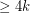has a homomorphism to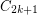.

This conjecture is Jaeger's modular orientation conjecture restricted to planar graphs and then dualized. To see this duality, first note that circular coloring and circular flows are dual for planar graphs, and then observe that-orientations are equivalent to-flows and-colorings are equivalent to homomorphisms to. So ifandare dual planar graphs, then we have the following equivalences.

\itemhas a-orientation. \itemhas a-flow. \itemhas a-coloring. \itemhas a homomorphism to.

There is an easy family of graphs which show that the above conjecture (if true) is best possible. Letbe the graph obtained from an odd circuit of lengthby adding a new vertexjoined to every existing vertex by a path of length. Now,is a planar graph of girth, but there is no homomorphism fromto. To see the latter claim, suppose (for a contradiction) that such a homomorphsimexists, letbe the unique circuit ofand let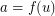. Now, no vertex incan map tosince every such vertex is distancefrom. However we must then have a homomorphism fromto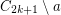, which is impossible sinceis an odd circuit and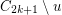is bipartite.

The k=1 case of the above conjecture asserts that every (loopless) triangle free planar graph has a homomorphism to the triangle. In other words, every (loopless) triangle free planar graph is 3-colorable. This is a well known theorem of Grotszch. For every k>1, the above conjecture is still open. Actually, I think this conjecture is already quite interesting for k=2. One reason is that this case of the conjecture implies the 5-color theorem for planar graphs. To see this implication, suppose that the above conjecture is true for k=2, let G be a simple loopless planar graph, and let G' be the graph obtained from G by subdividing each edge two times. Now, G' has girth at least 9, so by our assumption there is a homomorphism from G' to C_5. It is easy to see that adjacent vertices of G must map to different vertices of C_5 under this homomorphism. Thus, we have a proper 5-coloring of G as desired.

Let us call a homomorphism toa-coloring. It is quite easy to show that every planar graph of girth > 10k has a-coloring. This follows from a simple degeneracy argument: Every such (nonempty) graph must have a either a vertex of degree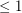, or a path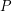of lengthall of whose internal vertices have degree two. Both of these configurations are reducible, in the sense that we may delete either a vertex of degreeor the interior vertices ofand then extend any-coloring of the resulting graph to a-coloring of the original. By more complicated, but similar degeneracy arguments, we can approach this conjecture. To my knowledge, the best result to date is as follows.

Theorem  (Borodin, Kim, Kostochka, West)   Every planar graph of girth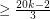has a homomorphism to.

For the special case of the conjecture when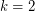, Matt DeVos and Adam Deckelbaum have an unpublished improvement showing that every planar graph with odd girthhas a homomorphism to.

## Bibliography

[BKKW] O. V. Borodin, S. J. Kim, A. V. Kostochka, D. B. West, Homomorphisms from sparse graphs with large girth. Dedicated to Adrian Bondy and U. S. R. Murty. J. Combin. Theory Ser. B 90 (2004), no. 1, 147--159. MathSciNet

[Ja] F. Jaeger, On circular flows in graphs in Finite and Infinite Sets, volume 37 of Colloquia Mathematica Societatis Janos Bolyai, edited by A. Hajnal, L. Lovasz, and V.T. Sos. North-Holland (1981) 391-402.

[Zh] X. Zhu, Circular chromatic number of planar graphs of large odd girth, Electronic Journal of Combinatorics Vol. 8 no. 1 (2001).

* indicates original appearance(s) of problem.